# Fire Case - Liquid Filled Vessel - Intergraph Smart Instrumentation - 13.1 - Reference - Hexagon PPM

## Integraph Smart Instrumentation Sizing Equations

Language
English (United States)
Product
Intergraph Smart Instrumentation
Search by Category
Reference
Smart Instrumentation Version
13.1
1. Calculate maximum discharge: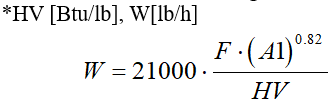2. Calculate upstream relieving pressure: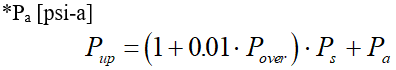3. Calculate Total back pressure: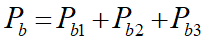4. Calculate Critical flow throat pressure: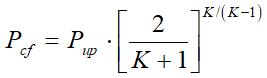5. Effective coefficient of discharge: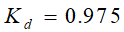.

6. The condition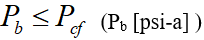determines:

Case A - Critical flow

1. Calculate Coefficient for critical flow equation: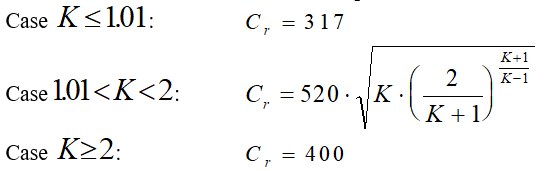2. Calculate Capacity correction factor due to back pressure (if Kb is not entered by user).

1. For conventional and Pilot operated valves,.Kb =1.

2. For Bellows valve: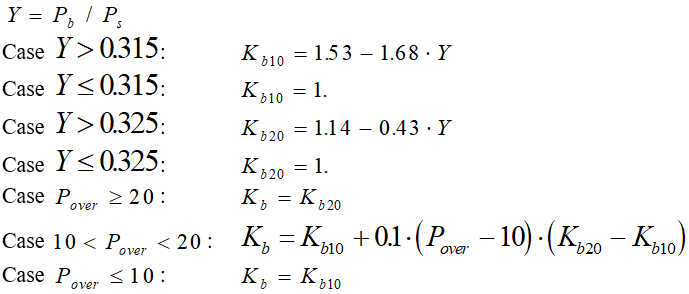3. Estimate Required effective discharge area: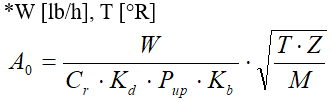Case B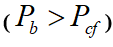- Sub-critical flow: Bellows Valve.

1. Calculate Cr: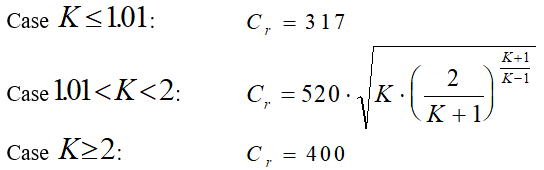2. If Capacity correction factor due to back pressure is not entered by user, Kb =1.

3. Estimate Required effective discharge area: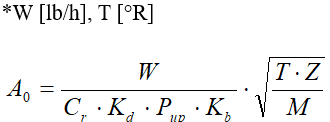Case c- Sub-critical flow: Conventional and Pilot operated valves..

1. Calculate Coefficient for sub-critical flow equation: *Pb [psi-a]

1.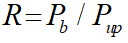2.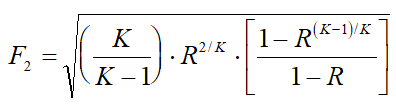2. Estimate Required effective discharge area: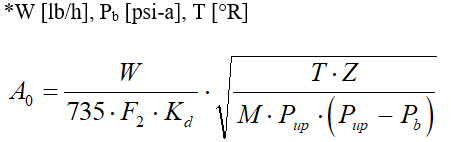7. For Rupture disk application, calculate Required effective discharge area:

A = A0 / Kcc